# How to Calculate and Solve for Normal Stress | Rock Mechanics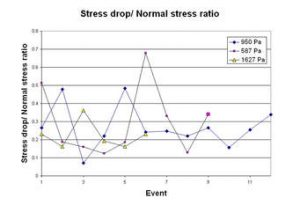The image above represents normal stress.

To calculate for normal stress, two essential parameters are needed and these parameters are normal force (ΔN) and area (ΔA).

The formula for calculating the normal stress:

σn = ΔN / ΔA

Where;

σn = Normal Stress
ΔN = Normal Force
ΔA = Area

Let’s solve an example;
Calculate the normal stress with a normal force of 12 and an area of 22.

This implies that;

ΔN = Normal Force = 12
ΔA = Area = 22

σn = ΔN / ΔA
σn = 12 / 22
σn = 0.54

Therefore, the normal stress is 0.54 Pa.

Calculating the Normal Force when the Normal Stress and Area is Given.

ΔN = σn x ΔA

Where;

ΔN = Normal Force
σn = Normal Stress
ΔA = Area

Let’s solve an example;
Calculate the normal force when the normal stress is 25 with an area of 10.

This implies that;

σn = Normal Stress = 25
ΔA = Area = 10

ΔN = σn x ΔA
ΔN = 25 x 10
ΔN = 250

Therefore, the normal force is 250.

Calculating the Area when the Normal Stress and Normal Force is Given.

ΔA = ΔN / σn

Where;

ΔA = Area
ΔN = Normal Force
σn = Normal Stress

Let’s solve an example;
Given that the normal stress is 20 and the normal force is 240. Find the area?

This implies that;

σn = Normal Stress = 20
ΔN = Normal Force = 240

ΔA = ΔN / σn
ΔA = 240 / 20
ΔA = 12

Therefore, the area is 12.

Nickzom Calculator – The Calculator Encyclopedia is capable of calculating the normal stress.

To get the answer and workings of the normal stress using the Nickzom Calculator – The Calculator Encyclopedia. First, you need to obtain the app.

You can get this app via any of these means:

To get access to the professional version via web, you need to register and subscribe for NGN 1,500 per annum to have utter access to all functionalities.
You can also try the demo version via https://www.nickzom.org/calculator

Apple (Paid) – https://itunes.apple.com/us/app/nickzom-calculator/id1331162702?mt=8
Once, you have obtained the calculator encyclopedia app, proceed to the Calculator Map, then click on Geology under Add-on.Now, Click on Rock Mechanics under GeologyNow, Click on Normal Stress under Rock Mechanics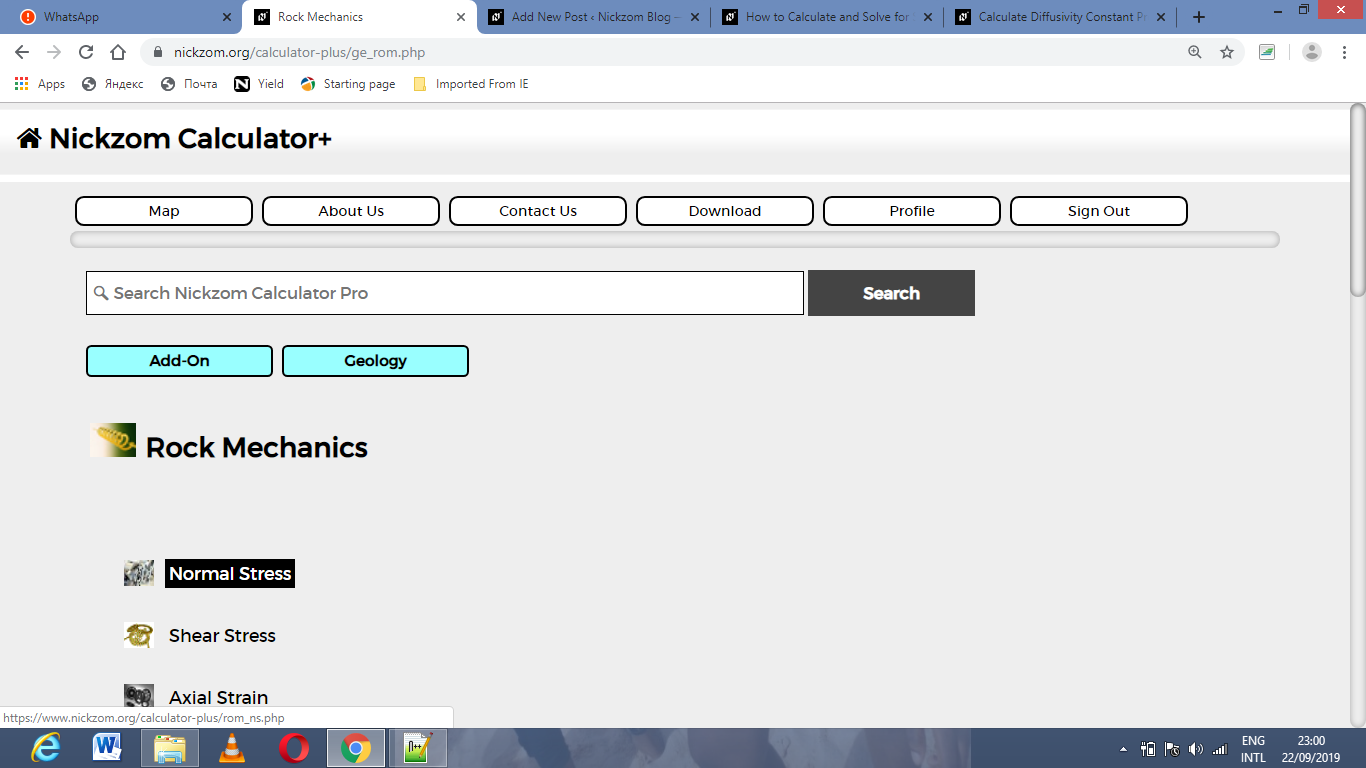The screenshot below displays the page or activity to enter your values, to get the answer for the normal stress according to the respective parameters which are the normal force (ΔN) and area (ΔA).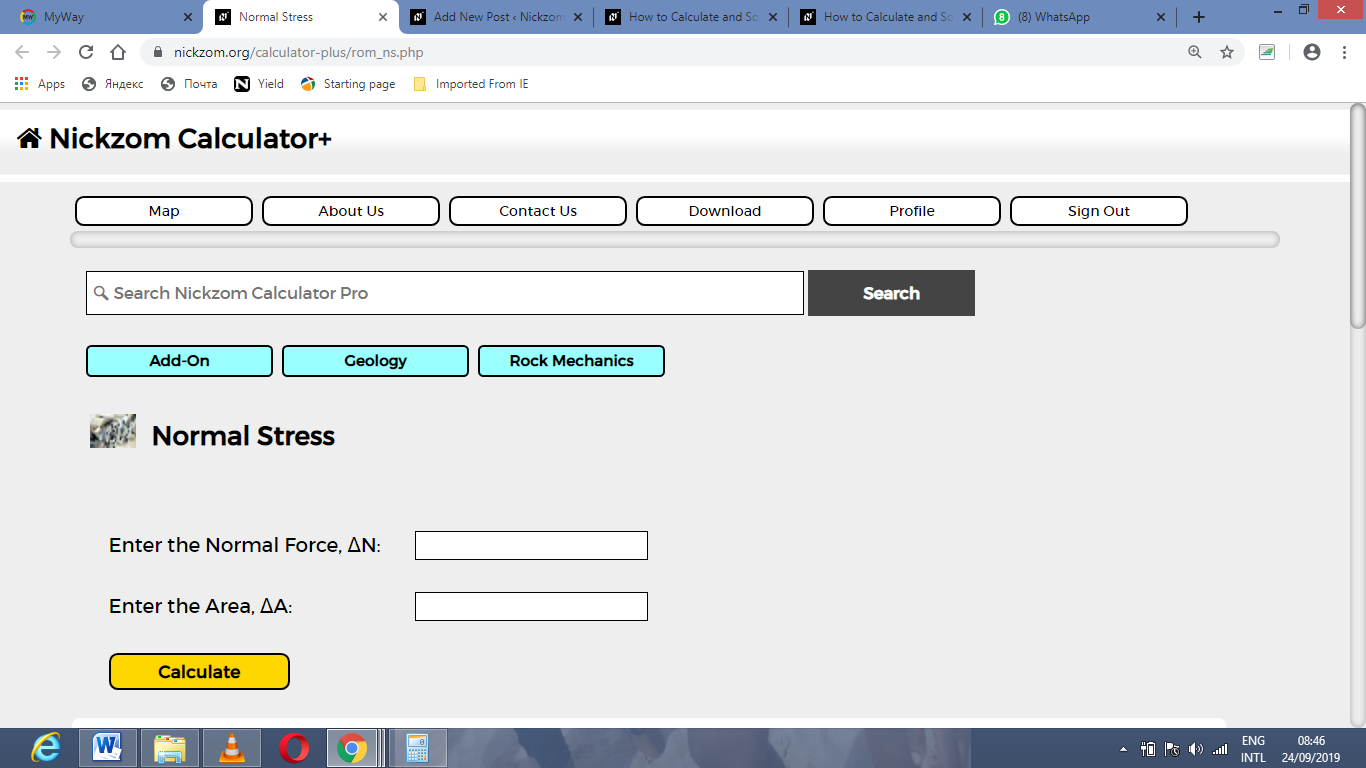Now, enter the values appropriately and accordingly for the parameters as required by the normal force (ΔN) is 12 and area (ΔA) is 22.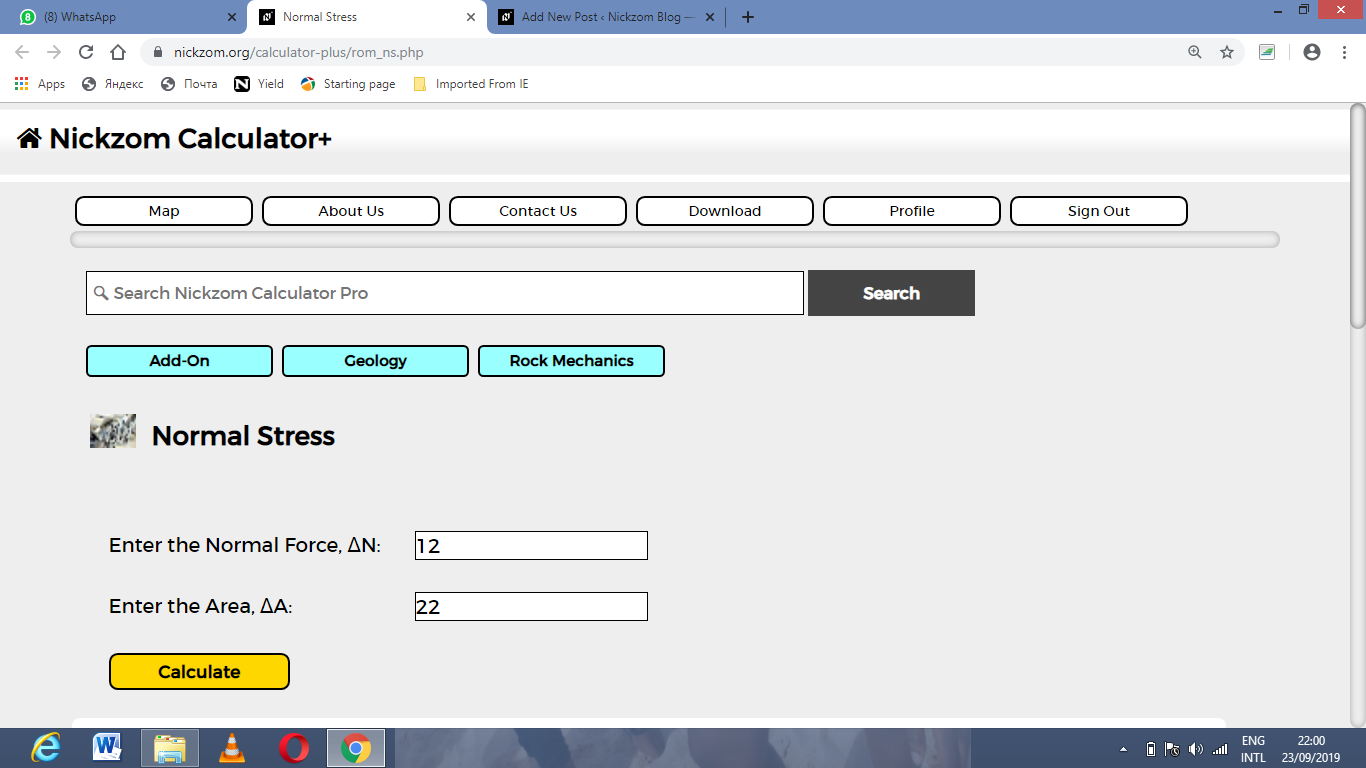Finally, Click on Calculate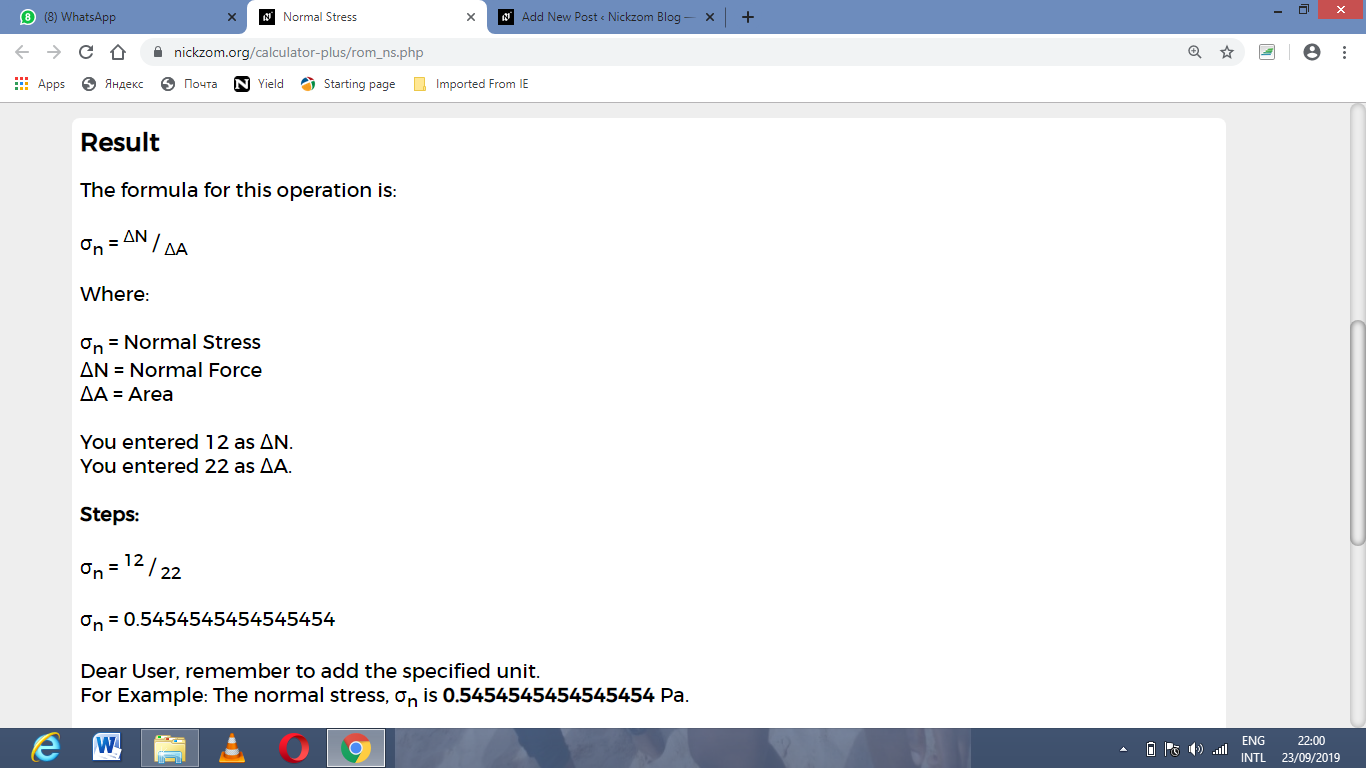As you can see from the screenshot above, Nickzom Calculator– The Calculator Encyclopedia solves for the normal stress and presents the formula, workings and steps too.